# Nonlinear Least-Squares

Knitro provides a specialized API for nonlinear least-squares models of the following form: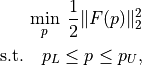where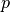is a parameter to be optimized and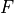is a differentiable function, which is called a residual. This type of problem appears very often in statistics, data-mining and machine learning. Using the nonlinear least-squares API, you are able to model a nonlinear least-squares problem in standard form above and use the Gauss-Newton Hessian option.

The Gauss-Newton Hessian provides a positive semi-definite Hessian approximation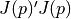(where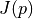is the Jacobian matrix of the residual functions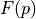) at every iteration and has good local convergence properties in practice. The Gauss-Newton Hessian option KN_HESSOPT_GAUSS_NEWTON, is the default Hessian option when using the nonlinear least-squares API. The quasi-Newton Hessian options are also available through the least-squares API, however, the user-supplied exact Hessian can only be specified using the standard API.

Any of the Knitro algorithms can be used through the least-squares API. Knitro will behave like a Gauss-Newton method by using the linesearch methods algorithm = KN_ALG_BAR_DIRECT or KN_ALG_ACT_SQP, and will be very similar to the classical Levenberg-Marquardt method when using the trust-region methods algorithm = KN_ALG_BAR_CG or KN_ALG_ACT_CG.

Residuals are added to a least-squares model using the KN_add_rsds(). The coefficients and sparsity structure for linear residuals (or linear terms inside nonlinear residuals) can be provided to Knitro throught the API function KN_add_rsd_linear_struct(). Constants can be added to residuals through KN_add_rsd_constants(). The nonlinear residuals and Jacobian are provided to Knitro using the callback functions KN_add_lsq_eval_callback() and KN_set_cb_rsd_jac() described below. Each user callback routine should return an int value of 0 if successful, or a negative value to indicate that an error occurred during execution of the user-provided function. If a callback function to evaluate the residual Jacobian is not provided, Knitro will approximate it using finite-differences. Please see Callable library API reference for more details on these API functions.

/** Add an evaluation callback for a least-squares models.  Similar to KN_add_eval_callback()
*  but for least-squares models.
*
*    nR            - number of residuals evaluated in the callback
*    indexRsds     - (length nR) index of residuals evaluated in the callback
*    rsdCallback   - a pointer to a function that evaluates any residual parts
*                    (specified by nR and indexRsds) involved in this callback
*    cb            - (output) the callback structure that gets created by
*                    calling this function; all the memory for this structure is
*                    handled by Knitro
*
*  After a callback is created by "KN_add_lsq_eval_callback()", the user can then
*  specify residual Jacobian information and structure through "KN_set_cb_rsd_jac()".
*  If not set, Knitro will approximate the residual Jacobian.  However, it is highly
*  recommended to provide a callback routine to specify the residual Jacobian if at all
*  possible as this will greatly improve the performance of Knitro.  Even if a callback
*  for the residual Jacobian is not provided, it is still helpful to provide the sparse
*  Jacobian structure for the residuals through "KN_set_cb_rsd_jac()" to improve the
*  efficiency of the finite-difference Jacobian approximation.  Other optional
*  information can also be set via "KN_set_cb_*() functions as detailed below.
*
*  Returns 0 if OK, nonzero if error.
*/
int  KNITRO_API KN_add_lsq_eval_callback (      KN_context_ptr            kc,
const KNINT                     nR,
const KNINT            * const  indexRsds,
KN_eval_callback * const  rsdCallback,
CB_context_ptr   * const  cb);

/** This API function is used to set the residual Jacobian structure and also
*  (optionally) a callback function to evaluate the residual Jacobian provided
*  through this callback.
*
*    cb               - a callback structure created from a previous call to
*    nnzJ             - number of nonzeroes in the sparse residual Jacobian
*                       computed through this callback; set to KN_DENSE_ROWMAJOR to
*                       provide the full Jacobian in row major order (i.e. ordered
*                       by rows/residuals), or KN_DENSE_COLMAJOR to provide the full
*                       Jacobian in column major order (i.e. ordered by columns/
*                       variables)
*    jacIndexRsds     - (length nnzJ) residual index (row) of each nonzero;
*                       set to NULL if nnzJ=KN_DENSE_ROWMAJOR/KN_DENSE_COLMAJOR or nnzJ=0
*    jacIndexVars     - (length nnzJ) variable index (column) of each nonzero;
*                       set to NULL if nnzJ=KN_DENSE_ROWMAJOR/KN_DENSE_COLMAJOR or nnzJ=0
*    rsdJacCallback   - a pointer to a function that evaluates any residual Jacobian
*                       parts involved in this callback; set to NULL if using a finite-
*                       difference Jacobian approximation (specified via KN_set_cb_gradopt())
*
*  The user should generally always try to define the sparsity structure
*  for the Jacobian ("nnzJ", "jacIndexRsds", "jacIndexVars").  Even when
*  using a finite-difference approximation to compute the Jacobian, knowing the
*  sparse structure of the Jacobian can allow Knitro to compute this
*  finite-difference approximation faster.  However, if the user is unable to
*  provide this sparsity structure, then one can set "nnzJ" to KN_DENSE_ROWMAJOR or
*  KN_DENSE_COLMAJOR and set "jacIndexRsds" and "jacIndexVars" to NULL.
*/
int  KNITRO_API KN_set_cb_rsd_jac (      KN_context_ptr            kc,
CB_context_ptr            cb,
const KNLONG                    nnzJ, /* or KN_DENSE_* */
const KNINT            * const  jacIndexRsds,
const KNINT            * const  jacIndexVars,
KN_eval_callback * const  rsdJacCallback); /* nullable *


There is currently no callback for the exact Hessian in the least-squares API. If you wish to provide a callback for the user-supplied exact Hessian, you must use the standard API.

After solving, the residuals and residual Jacobian can be retrieved through the API functions KN_get_rsd_values() and KN_get_rsd_jacobian_values(). See Callable library API reference for more details.

## C example

The following C example illustrates how to use the Knitro least squares interface.

/*  A simple nonlinear least-squares problem with 6 residual functions:
*
*  min   ( x0*1.309^x1 - 2.138 )^2 + ( x0*1.471^x1 - 3.421 )^2
*         + ( x0*1.49^x1 - 3.597 )^2 + ( x0*1.565^x1 - 4.34 )^2
*         + ( x0*1.611^x1 - 4.882 )^2 + ( x0*1.68^x1-5.66 )^2
*/

#include <stdio.h>
#include <stdlib.h>
#include <math.h>

#include "knitro.h"

int callbackEvalR (KN_context_ptr             kc,
CB_context_ptr             cb,
KN_eval_request_ptr const  evalRequest,
KN_eval_result_ptr  const  evalResult,
void              * const  userParams)
{
const double *x;
double *rsd;

if (evalRequest->type != KN_RC_EVALR)
{
printf ("*** callbackEvalR incorrectly called with eval type %d\n",
evalRequest->type);
return( -1 );
}
x = evalRequest->x;
rsd = evalResult->rsd;

/** Evaluate nonlinear residual components */
rsd = x * pow(1.309, x);
rsd = x * pow(1.471, x);
rsd = x * pow(1.49, x);
rsd = x * pow(1.565, x);
rsd = x * pow(1.611, x);
rsd = x * pow(1.68, x);

return( 0 );
}

int callbackEvalRJ (KN_context_ptr             kc,
CB_context_ptr             cb,
KN_eval_request_ptr const  evalRequest,
KN_eval_result_ptr  const  evalResult,
void              * const  userParams)
{
const double *x;
double *rsdJac;

if (evalRequest->type != KN_RC_EVALRJ)
{
printf ("*** callbackEvalRJ incorrectly called with eval type %d\n",
evalRequest->type);
return( -1 );
}
x = evalRequest->x;
rsdJac = evalResult->rsdJac;

/** Evaluate non-zero residual Jacobian elements (row major order). */
rsdJac = pow(1.309, x);
rsdJac = x * log(1.309) * pow(1.309, x);
rsdJac = pow(1.471, x);
rsdJac = x * log(1.471) * pow(1.471, x);
rsdJac = pow(1.49, x);
rsdJac = x * log(1.49) * pow(1.49, x);
rsdJac = pow(1.565, x);
rsdJac = x * log(1.565) * pow(1.565, x);
rsdJac = pow(1.611, x);
rsdJac = x * log(1.611) * pow(1.611, x);
rsdJac = pow(1.68, x);
rsdJac = x * log(1.68) * pow(1.68, x);

return( 0 );
}

int  main (int  argc, char  *argv[])
{
/** Declare variables. */
KN_context   *kc;
int     i, error;
int     n, m;
/** Used to set constants for residuals */
double  constants = {-2.138, -3.421, -3.597, -4.34, -4.882, -5.66};
/** Pointer to structure holding information for evaluation
*  callbacks. */
CB_context   *cb;
/** Solution information. */
int     nRC, nStatus;
double  x;
double  obj;

/** Create a new Knitro solver instance. */
error = KN_new(&kc);
if (error) exit(-1);
if (kc == NULL)
{
printf ("Failed to find a valid license.\n");
return( -1 );
}

*  Note: Any unset lower bounds are assumed to be
*  unbounded below and any unset upper bounds are
*  assumed to be unbounded above. */
n = 2; /* # of variables/parameters */
if (error) exit(-1);

m = 6; /* # of residuals */
if (error) exit(-1);

/** Set the array of constants in the residuals */
if (error) exit(-1);

/** Add a callback function "callbackEvalR" to evaluate the nonlinear
*  residual components.  Note that the constant terms are added
*  separately above, and will not be included in the callback. */
error = KN_add_lsq_eval_callback_all (kc, callbackEvalR, &cb);
if (error) exit(-1);

/** Also add a callback function "callbackEvalRJ" to evaluate the
*  Jacobian of the residuals.  If not provided, Knitro will approximate
*  the residual Jacobian using finite-differencing.  However, we recommend
*  providing callbacks to evaluate the exact Jacobian whenever
*  possible as this can drastically improve the performance of Knitro.
*  We specify the residual Jacobian in "dense" row major form for simplicity.
*  However for models with many sparse residuals, it is important to specify
*  the non-zero sparsity structure of the residual Jacobian for efficiency
*  (this is true even when using finite-difference gradients). */
error = KN_set_cb_rsd_jac (kc, cb, KN_DENSE_ROWMAJOR, NULL, NULL, callbackEvalRJ);
if (error) exit(-1);

/** Solve the problem.
*
*  Return status codes are defined in "knitro.h" and described
*  in the Knitro manual.
*/
nRC = KN_solve (kc);

/** Delete the knitro solver instance. */
KN_free (&kc);

return( 0 );
}